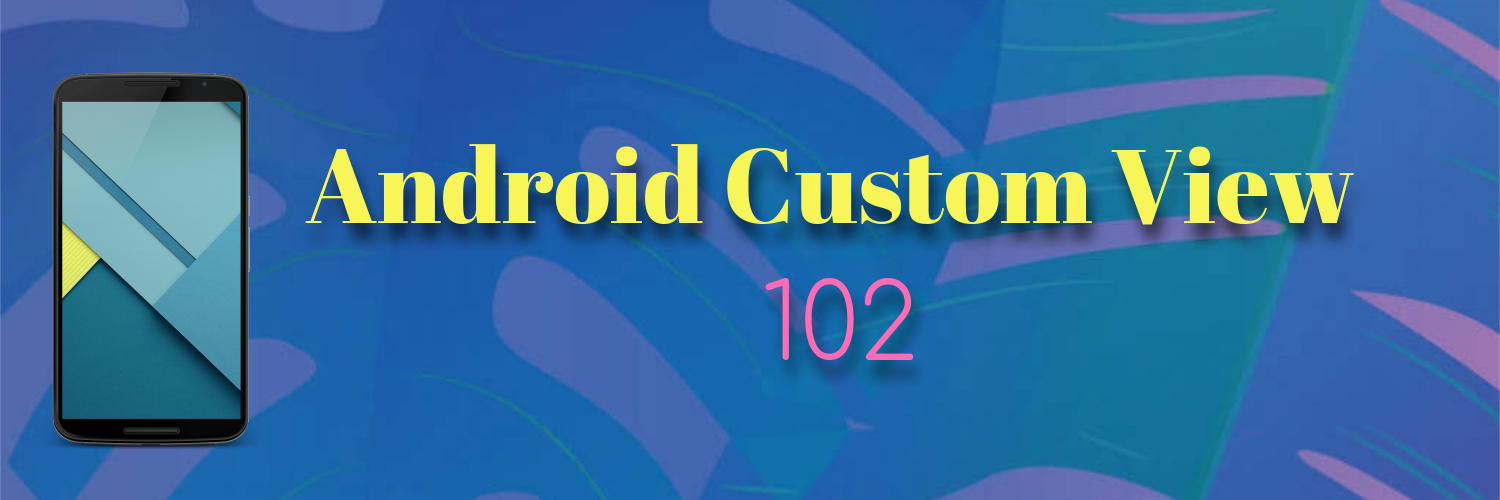# Android Custom View 102 (Part I)

The basics of drawing stuffIf 101 is like a crash course, then this 102 will provide much more detail.

In this 1st part, we focus on only one thing: how to draw things.

# Draw Color

Draw the entire view with one color.

``````public class DrawColorView extends View {

...

@Override
protected void onDraw(Canvas canvas) {
super.onDraw(canvas);
canvas.drawColor(Color.YELLOW);
}
}
``````

# Draw Circle

Draw a circle on the view.

Pay attention that all `drawXXX()` functions use pixel as unit. But we can convert dp to px with this utils function.

``````public class Utils {

public static float convertDpToPx(Context context, float dp) {
return dp * context.getResources().getDisplayMetrics().density;
}
}
``````
``````public class DrawCircleView extends View {

...

paint1 = new Paint();
paint1.setAntiAlias(true);
cx1 = convertDpToPx(context, 50);
cy1 = convertDpToPx(context, 50);

paint2 = new Paint();
paint2.setAntiAlias(true);
paint2.setStyle(Paint.Style.STROKE);
cx2 = convertDpToPx(context, 120);
cy2 = convertDpToPx(context, 50);

paint3 = new Paint();
paint3.setAntiAlias(true);
paint3.setColor(Color.BLUE);
cx3 = convertDpToPx(context, 190);
cy3 = convertDpToPx(context, 50);

paint4 = new Paint();
paint4.setAntiAlias(true);
paint4.setStyle(Paint.Style.STROKE);
paint4.setStrokeWidth(convertDpToPx(context, 10));
cx4 = convertDpToPx(context, 260);
cy4 = convertDpToPx(context, 50);

...

@Override
protected void onDraw(Canvas canvas) {
super.onDraw(canvas);
}
}
``````

The above code draws 4 circles in the view:

This is simple, `cx`, `cy` and `radius` determine the position of the circle and how big it is. The `paint` determines the styles.

# Draw Rectangle

Draw a rectangle in the view.

``````public class DrawRectView extends View {

...

@Override
protected void onDraw(Canvas canvas) {
super.onDraw(canvas);
canvas.drawRect(left, top, right, bottom, paint);
}
}
``````

# Draw Point

Draw a point in the view.

``````public class DrawPointView extends View {

...

float strokeWidth = convertDpToPx(context, 20);

paint1 = new Paint();
paint1.setStrokeWidth(strokeWidth);
paint1.setStrokeCap(Paint.Cap.ROUND);

paint2 = new Paint();
paint2.setStrokeWidth(strokeWidth);
paint2.setStrokeCap(Paint.Cap.BUTT);

x1 = convertDpToPx(context, 100);
y1 = convertDpToPx(context, 100);

x2 = convertDpToPx(context, 200);
y2 = convertDpToPx(context, 100);

...

@Override
protected void onDraw(Canvas canvas) {
super.onDraw(canvas);
canvas.drawPoint(x1, y1, paint1);
canvas.drawPoint(x2, y2, paint2);
}
}
``````

The shape of the point is determined by the `paint`’s `Cap`. There are 3 values: BUTT, ROUND and SQUARE. Both `BUTT` and `SQUARE` will draw a square point, `ROUND` will draw a round point.

There are many other methods that can draw different shapes, like `drawArc()`, `drawOval`, etc. The usages of them are similar, so I will not talk them in detail here. But there is one more complex method called `drawPath()` which is worth mentioning.

# Draw Path

Using path we can draw any shape.

Let’s try something simple.

``````path.lineTo(100, 100);
path.rLineTo(100, 0);
canvas.drawPath(path, paint);
``````

`lineTo(x, y)` will draw a line from `(0, 0)` to `(x, y)`. Then, `rLineTo(dx, dy)` means take the current `(x, y)`, draw a line to (`x + dx, y + dy)`. `r` means relative.

Now let’s try combine a line with an arc.

``````@Override
protected void onDraw(Canvas canvas) {
super.onDraw(canvas);
Path path = new Path();
path.lineTo(100, 100);
path.arcTo(100, 100, 300, 300, -90, 90, true);
canvas.drawPath(path, paint);
}
``````

We can see that there are two parts, the line and the arc. But what is about the last param which now is set to true in `arcTo()`? It is `forceMoveTo`. The easiest way to understand this is think of the drawing process as drawing with a pen. When the line and arc are seperate, after you draw the line, there are two ways to draw the arc: lift up and move the pen to the starting point of the arc and draw it, or drag the pen to the starting point of the arc and draw it. Let’s try to set it to `false` and see what it looks like:

So if `forceMoveTo` equals true, the pen is lifted. Otherwise the pen is dragged.

# Draw Heart

OK, let’s try to draw a heart.

``````@Override
protected void onDraw(Canvas canvas) {
super.onDraw(canvas);
path.addArc(200, 200, 400, 400, -225, 225);
path.arcTo(400, 200, 600, 400, -180, 205, false);
path.lineTo(400, 542);
canvas.drawPath(path, paint);
}
``````

This concludes part 1, in part 2 we will take a closer look at the `paint` class.

To be continued …